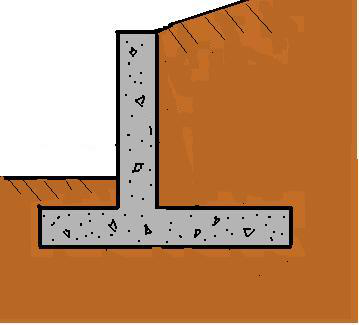* Log In to use the Calculate function * Become a Member!

###### Reinforced Concrete Cantilever Retaining Walls:Retaining Wall Design
• Calculate active earth pressure coefficient, earth pressure, horizontal resultant and moment.

• Calculate the earth pressure horizontal resultant from a uniform load surcharge.
• Calculate the earth pressure horizontal resultant from a point load surcharge.
• Calculate the earth pressure horizontal resultant from a strip load surcharge.
• Calculate the earth pressure horizontal resultant from a ramp load surcharge.
• Calculate the earth pressure horizontal resultant from a triangle load surcharge.
• Calculate the earth pressure horizontal resultant from a line load surcharge.
• Calculate the slip plane angle of the soil for the soil pressure wedge calculation, directly for noncohesive soils and by trial and error for cohesive soils.
Wedge method
• Calculate soil pressure resultant with or without a surface surcharge by the approximate wedge method.
• Create a concrete cantilever retaining wall trial design section.
• Calculate the resistance to sliding for a retaining wall without a keyed base.
• Calculate the resistance to sliding for a retaining wall with a keyed base.
• Calculate the eccentricity, maximum and minimum soil pressure beneath the base of a retaining wall.
Bearing pressure resultants
• Calculate the bearing pressure resultants for the toe and heel of a concrete cantilever retaining wall to be used in the reinforcement calculations.
• Check the bearing capacity of a concrete cantilever retaining wall.
• Calculate the distance to steel and the area of steel reinforcement for the stem section of a concrete cantilever retaining wall.
• Calculate the distance to steel and the area of steel reinforcement for the heel section of the base of a concrete cantilever retaining wall.
• Calculate the distance to steel and the area of the steel reinforcement for the toe section of the base of a concrete cantilever retaining wall.
• Calculate the development length for the steel reinforcement of the stem section of a concrete cantilever retaining wall.
• Calculate the lateral earth force acting on a retaining wall using the wedge method.
• Calculate the lateral earth force acting on a retaining wall using the Rankine Formula.
• Check the sliding resistance for a retaining wall.
• Check the resistance to sliding, the turnover stability, the bearing capacity and calculate the required areas of steel reinforcement for a proposed retaining wall.

 Start Using The Calculations >>> Become A Member Now!